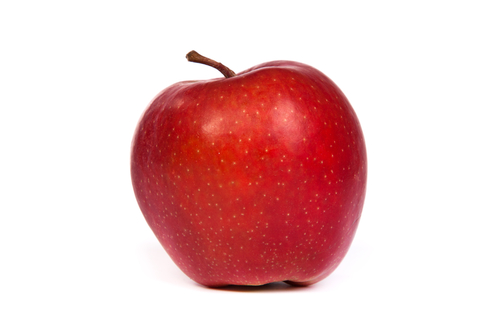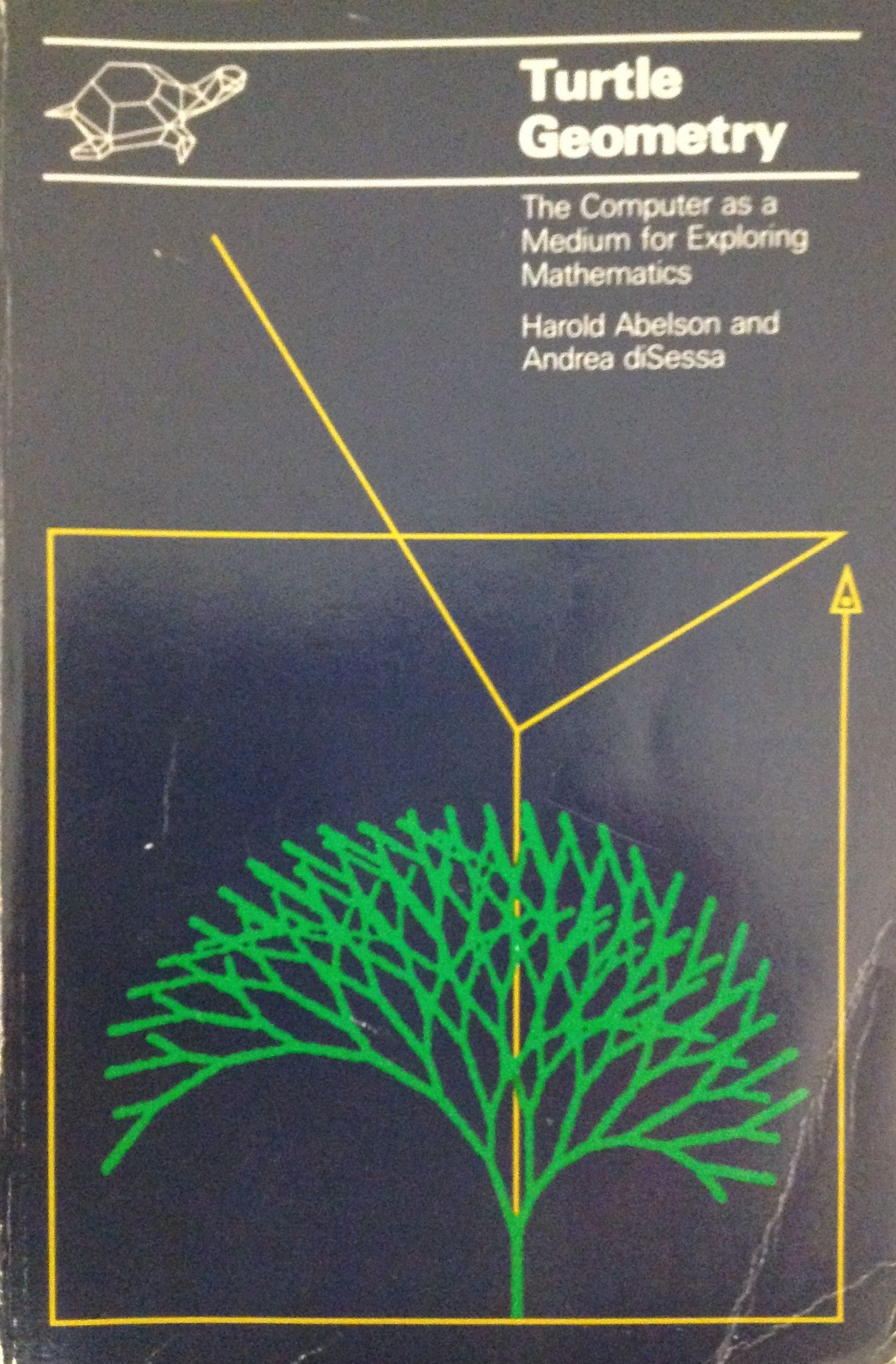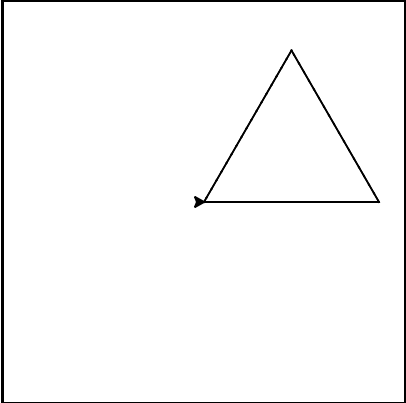# 17.6.Teacher Note: Turtle Geometry¶

The turtle is actually useful for exploring a wide variety of ideas in geometry. The book Turtle Geometry does a wonderful job of showing how turtles can be used to explore a wide variety of geometric, mathematical, and scientific ideas (e.g., using turtles to model insect behavior). The example pattern below is drawn from that book.Figure 1: A scan of Mark’s copy of the book

# 17.7. Total Turtle Trip Theorem¶

That last piece of code is actually a pattern for a wide variety of geometric shapes. Here’s a triangle. It may not be obvious why we turn 120 in this program, but it will be soon.

Run the code to see what it draws.

And here’s a pentagon.

Run the code to see what it draws.

The Total Turtle Trip Theorem states that the turtle will draw a closed figure with n sides when the sum of the angles turned is a multiple of 360. In the triangle example `3 * 120 = 360` and in the pentagon example `5 * 72 = 360`.

Change the `??` in line 7 below to the amount to turn each time to draw a 12 sided polygon, which is called a dodecagon. If you get it right the turtle will draw a 12 sided closed polygon.

You will need to replace the ‘??’ on line 7 to have the code correctly draw a dodecagon (a polygon with 12 sides).

The following program uses a turtle to draw a triangle as shown below, but the lines are mixed up. The program should do all necessary set-up and create the turtle. After that, iterate (loop) 3 times, and each time through the loop the turtle should go forward 100 pixels, and then turn left 120 degrees. Drag the needed blocks of statements from the left column to the right column and put them in the right order with the correct indention. There may be additional blocks that are not needed in a correct solution. Click on Check to see if you are right. You will be told if any of the lines are in the wrong order or are the wrong blocks.Write a function which takes in a turtle object and the number of sides as parameters to draw a polygon. Call the function to test it.

Run the code to see what it draws.